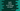# Swift program to check if two strings are equal or not## Check if two strings are equal or not in Swift

This swift tutorial will show you how to check if two strings are equal or not. I will show you two different ways to do a equal compare.

1. By using ==
2. By using elementsEqual

## Example of == :

This is the easiest way to compare two strings. == or equal-to operator. This operator is defined as below :

``static func == (String, String) -> Bool``

It takes two strings and compares them. Based on the result, it returns one boolean value indicating the strings are equal or not.

Let’s take a look at the below example :

``````let firstString = "Hello World"
let secondString = "HEllo World"
let thirdString = "Hello World"

print(firstString == secondString)
print(firstString == thirdString)``````

We have three stings here, but only firstString is equal to thirdString. If you run this code, it will print the below output :

``````false
true``````

## Example of elementsEqual :

elementsEqual is another method to compare two strings. It is used to compare two sequences. It returns one boolean value indicating whether both sequences contain the same elements in the same order.

This method is defined as below :

``func elementsEqual<OtherSequence>(OtherSequence) -> Bool``

Let’s try this method with the above strings :

``````let firstString = "Hello World"
let secondString = "HEllo World"
let thirdString = "Hello World"

print(firstString.elementsEqual(secondString))
print(firstString.elementsEqual(thirdString))``````

It will print the same output.

## Case insensitive comparison :

Above examples are doing case sensitive comparisons. To do case insensitive comparison, we need to convert both strings to lowercase using lowercased() method :

``````let firstString = "Hello World"
let secondString = "HEllo World"
let thirdString = "Hello World"

print(firstString.lowercased().elementsEqual(secondString.lowercased()))
print(firstString.lowercased().elementsEqual(thirdString.lowercased()))``````

This will print true for both.

``````true
true``````